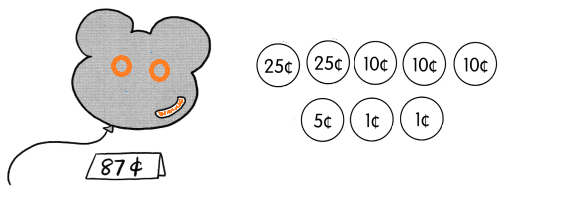# Math in Focus Grade 1 Chapter 19 Practice 3 Answer Key Counting Money

Go through the Math in Focus Grade 1 Workbook Answer Key Chapter 19 Practice 3 Counting Money to finish your assignments.

## Math in Focus Grade 1 Chapter 19 Practice 3 Answer Key Counting Money

Count on to find the value.

Example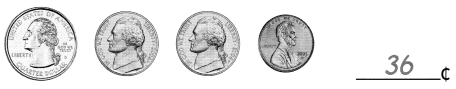Question 1.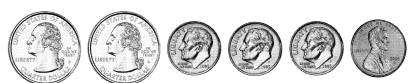___________¢
Explanation:
2 quarters + 3 dimes + 1 penny = 81¢
1 quarter = 25¢
1 dime = 10¢
1 penny = 1¢
25 + 25 + 10 + 10 + 10 + 1 = 81¢

Question 2.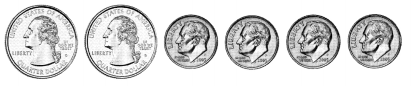___________¢
Explanation:
2 quarters + 4 dimes = 90¢
1 quarter = 25¢
1 dime = 10¢
25 + 25 + 10 + 10 +10 +10 = 90¢

Question 3.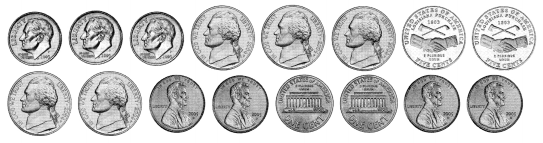___________¢
Explanation;
3 dimes + 7 nickels + 5 pennies = 70¢
1 dime = 10¢
1 nickel = 5¢
1 penny = 1¢
10 + 10 + 10 + 5 + 5 + 5 +5 + 5 + 5 + 5 + 1 + 1 + 1 + 1 + 1 = 70¢

Question 4.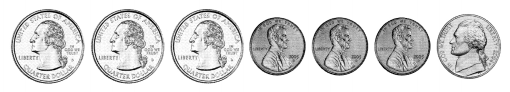___________¢
Explanation:
3 quarters + 3 pennies + 1 nickel = 83¢
1 quarter = 25¢
1 penny = 1¢
1 nickel = 5¢
25 + 25 + 25 + 1 + 1 + 1 + 5 = 83¢

Question 5.
Match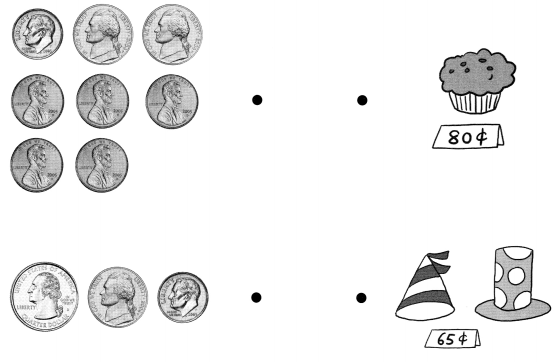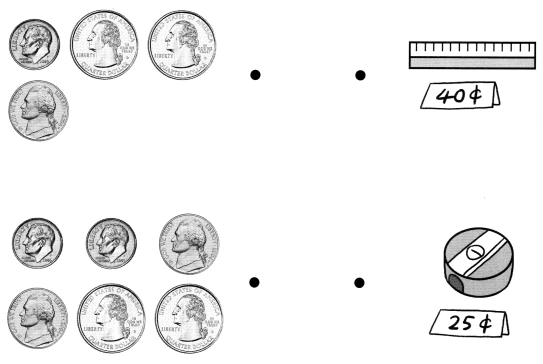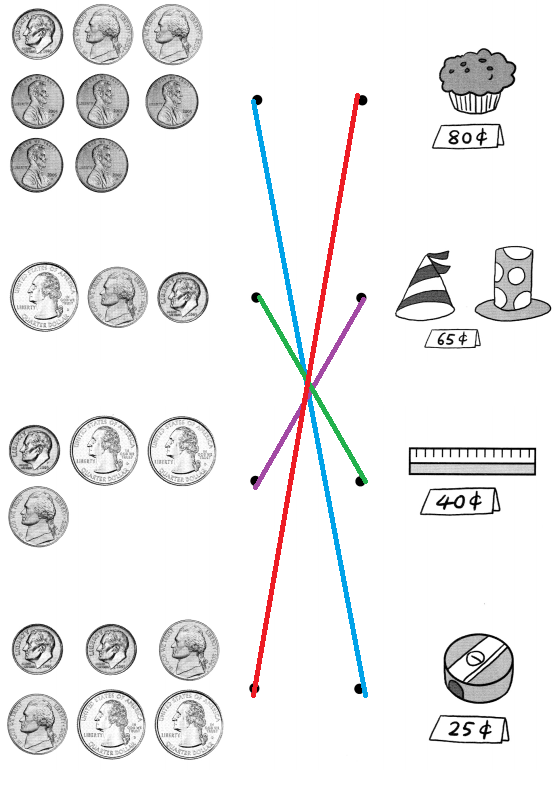Explanation:
1 quarter = 25¢
1 penny = 1¢
1 nickel = 5¢
1 dime = 10¢
80¢ = 2 dimes + 2 nickels + 2 quarters
65¢ = 1 dime + 2 quarters + 1 nickel
40¢ = 1 quarter + 1 nickel + 1 dime
25¢ = 1 dime + 2 nickels  + 5 pennies

Sort the coins.

Example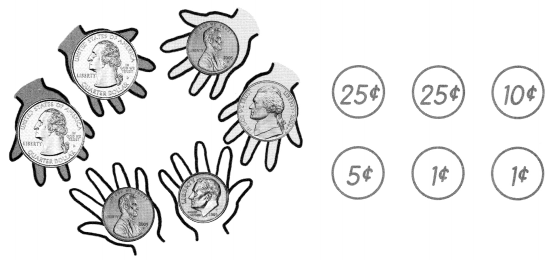Question 6.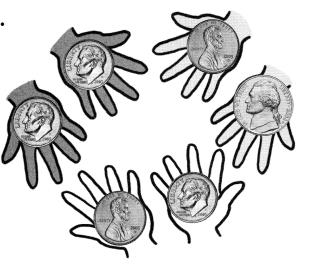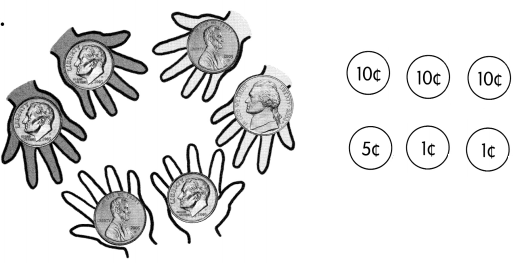Explanation:
The above given pictures of currency consists,
3 dimes, 1 nickel and 2 pennies.

Question 7.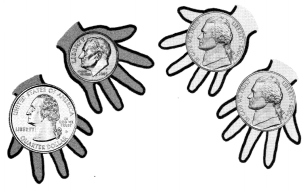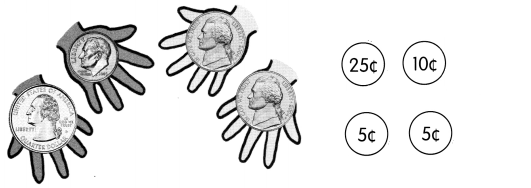Explanation:
The above given pictures of currency consists,
1 quarter, 1 dime, and 2 nickels.

Circle the coins you need to buy each thing.

Example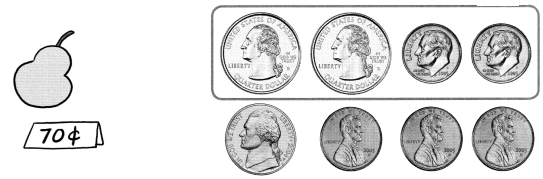Question 8.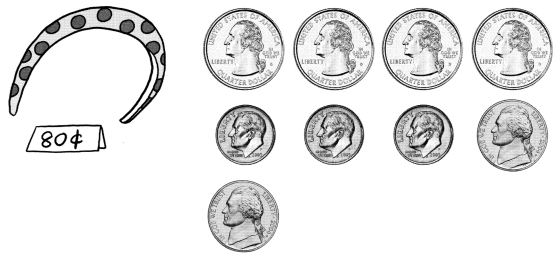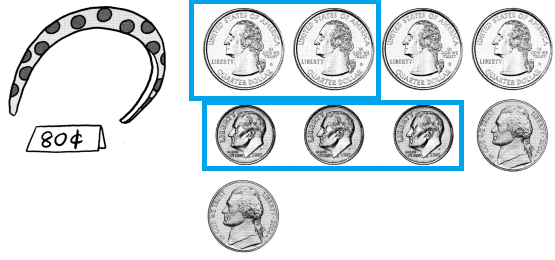Explanation:
The above picture costs  80¢
2 quarters + 3 dimes = 80¢
1 quarter = 25¢
1 dime = 10¢

Question 9.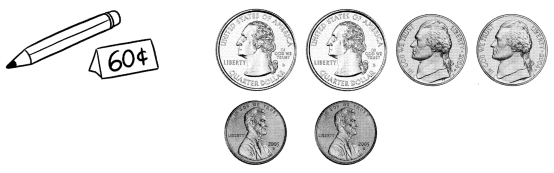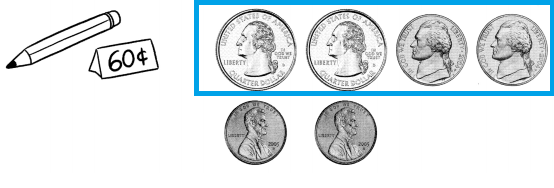Explanation:
Cost of pencil is 60¢
2 quarters + 2 nickels = 60¢
1 quarter = 25¢
1 nickel = 5¢

Question 10.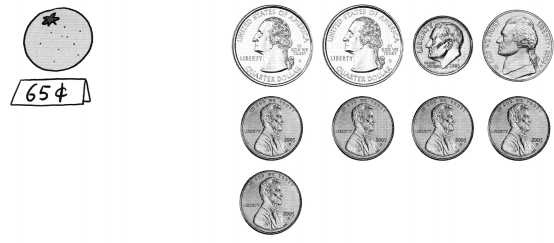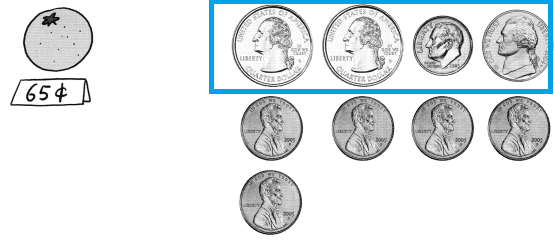Explanation:
Cost of Tomato 65¢
2 quarters + 1 dime + 1 nickel = 65¢
1 quarter = 25¢
1 dime = 10¢
1 nickel = 5¢

Question 11.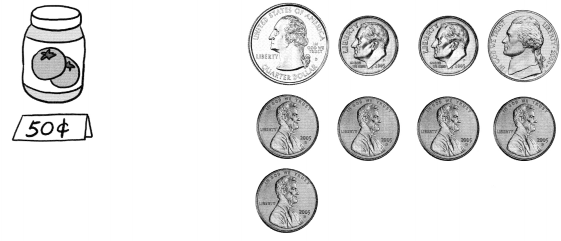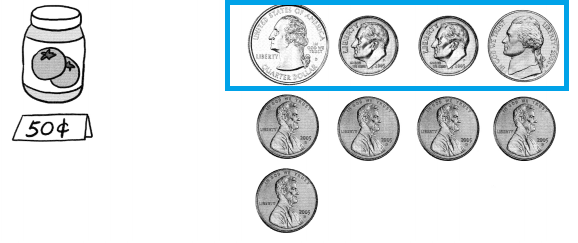Explanation:
Cost of 1 bottle is 50¢
1 quarter + 2 dimes + 1 nickel = 50¢
1 quarter = 25¢
1 dime = 10¢
1 nickel = 5¢

Question 12.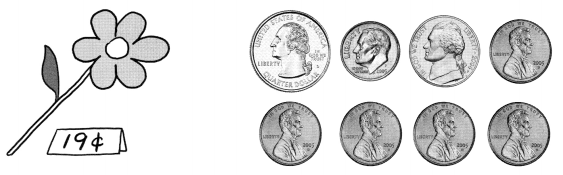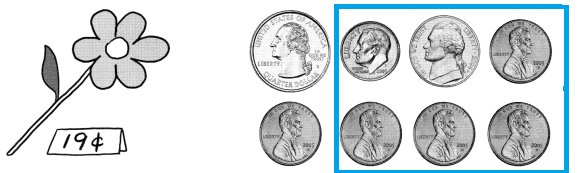Explanation:
Cost of flower is 19¢
1 dime + 1 nickel + 4 pennies = 19¢
1 penny = 1¢
1 dime = 10¢
1 nickel = 5¢

Question 13.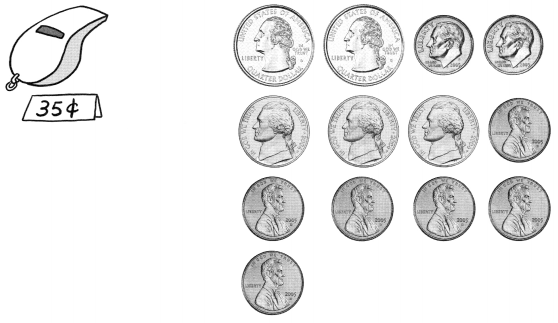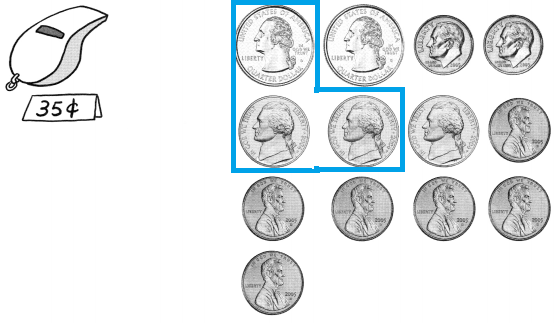Explanation:
Cost of Whistle is 35¢
1 quarter + 2 nickels = 35¢
1 quarter = 25¢
1 nickel = 5¢

Question 14.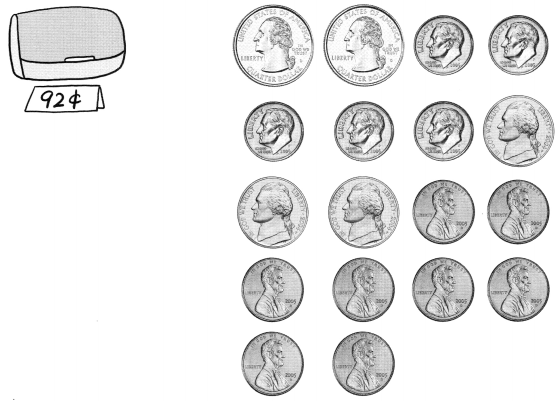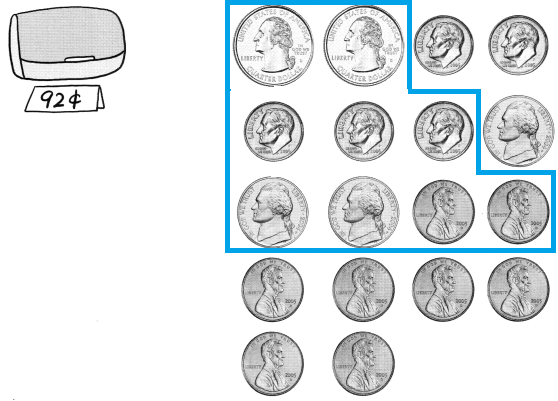Explanation:
Cost of Bag is 92¢
2 quarter + 3 dimes + 2 nickels + 2 pennies = 92¢
1 quarter = 25¢
1 dime = 10¢
1 nickel = 5¢
1 penny = 1¢

Complete the table.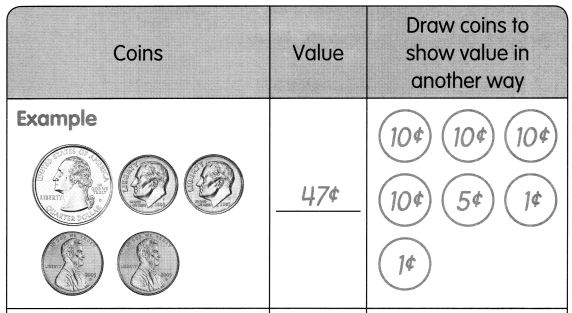Question 15.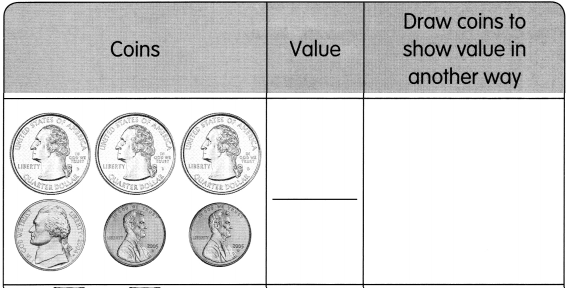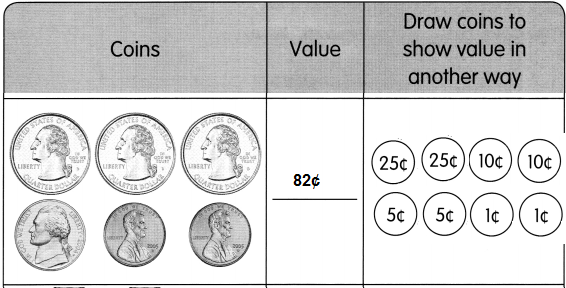Explanation:
In the table there are 3 quarters, 1 nickel and 2 pennies of coins are present.
1 quarter = 25¢
1 nickel = 5¢
1 penny = 1¢
25¢ + 25¢ + 25¢ + 5¢ + 5¢ + 1¢ + 1¢ = 82¢
82¢ can also be shown in another way as
2 quarters + 2 dimes + 2 nickels + 2 pennies = 82¢

Question 16.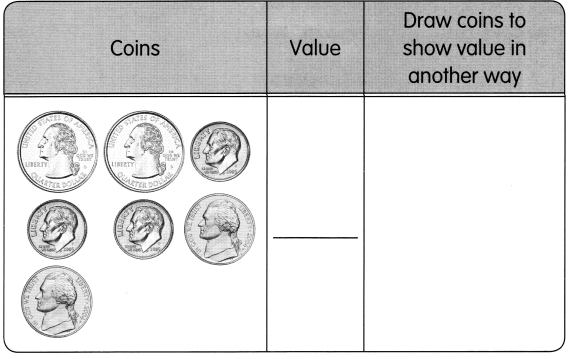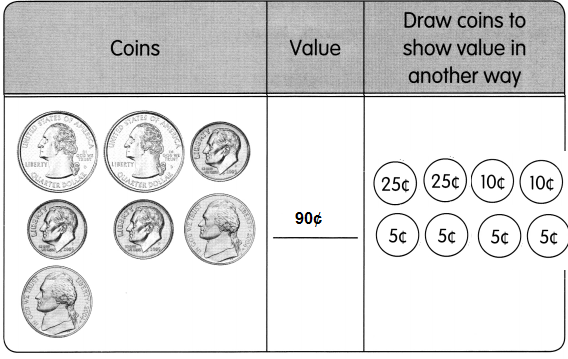Explanation:
In the table there are 2 quarters, 3 dimes and 2 nickels of coins are present.
1 quarter = 25¢
1 nickel = 5¢
1 dime = 10¢
25¢ + 25¢ + 10¢ + 10¢ + 10¢ + 5¢ + 5¢ = 90¢
90¢ can also be shown in another way as
2 quarters + 2 dimes + 4 nickels = 90¢

Use pennies 1¢, nickels 5¢, dimes 10¢, and quarters 25¢. Draw 2 ways to pay for the balloon.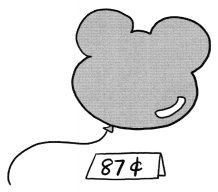Question 17.
25 + 25 + 10 + 10 + 5 + 5 + 5 + 1 + 1 = 87¢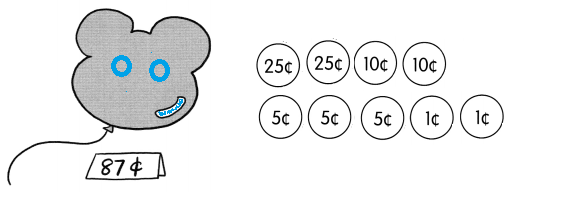Explanation:
1 quarter = 25¢
1 dime = 10¢
1 nickel = 5¢
1 penny = 1¢
2 quarters + 2 dimes + 3 nickels + 2 pennies
25 + 25 + 10 + 10 + 5 + 5 + 5 + 1 + 1 = 87¢

Question 18.